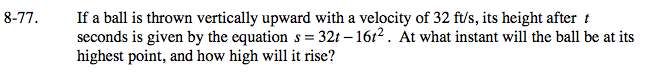### Home > PC > Chapter 8 > Lesson 8.2.1 > Problem8-77

8-77.

If a ball is thrown vertically upward with a velocity of 32 ft/s, its height after t seconds is given by the equation s = 32t −16t 2. At what instant will the ball be at its highest point, and how high will it rise? Homework Help ✎1. Set the function = 0.
2. Factor.
3. Solve for the t-intercepts.
4. Take the average of the x-intercepts to locate the t-coordinate of the vertex.
5. Substitute that t-value into the original equation to calculate the maximum height.